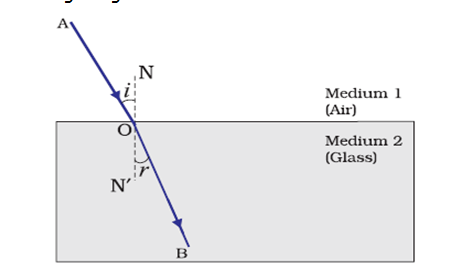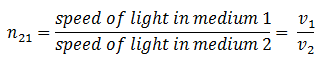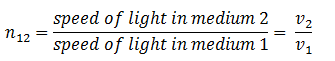# Laws of Refraction of light and Refractive index

## Laws of refraction of light(Snell�s law of refraction)

• Refraction is due to change in the speed of light as it enters from one transparent medium to another.
• Experiments show that refraction of light occurs according to certain laws.
• So Laws of refraction of light are
• The incident ray, the refracted ray and the normal to the interface of two transparent media at the point of incidence, all lie in the same plane.
• The ratio of sine of angle of incidence to the sine of angle of refraction is a constant, for the light of a given color and for the given pair of media. This law is also known as Snell�s law of refraction.
• If i is the angle of incidence and r is the angle of refraction then $\frac{\sin i} {\sin r} = constant = n$               (1)
This constant value is called the refractive index of the second medium with respect to the first.

## The Refractive Index

• We now know about refraction of light and the extent of the change in direction that takes place in a given pair of media is expressed in terms of the refractive index, the "constant" appearing in equation 1.
• The refractive index is related to an important physical quantity that is relative speed of propagation of light in different media as light propagates with different speeds in different media.
• Consider the figure given below• Let v1 be the speed of light in medium 1 and v2 be the speed of light in medium 2 then the refractive index of medium 2 with respect to medium 1 is given by the ratio of the speed of light in medium 1 and the speed of light in medium 2. So,where n21 is the refractive index of medium 2 with respect to medium 1.
• The refractive index of medium 1 with respect to medium 2 is represented as n12. It is given by• If medium 1 is vacuum or air, then the refractive index of medium 2 is considered with respect to vacuum. This is called the absolute refractive index of the medium.
• If c is the speed of light in the air and v is the speed of light in any medium then refractive index nm of the medium would be### Prove that the incident angle and the emergent angle in a rectangular glass slab are equal

• In the figure given above ABCD is a rectangular glass slab of thickness AD=BC=t. A ray PQ is incident on it an face AB at point Q, making an angle $PQN_1=i$, called angle of incidence.
• This ray refracts in the glass slab and goes along QR as refracted ray (as shown in the figure) and becomes incident on face DC at point R from inside the slab.
• $\angle RQ{{N}_{2}}=\angle QR{{N}_{3}}=r$ and is called angle of refraction.
• Now the ray emerges or comes out of the slab along RS making $\angle SR{{N}_{4}}=e$ , called the angle of emergence.
• This emergent ray is parallel to the incident ray. This can be proved as follows.
For refraction of Q :- from air to glass \begin{equation*} n=\frac{\sin i}{\sin r} \end{equation*} For refraction at R :- from glass to air \begin{equation} \frac{1}{n}=\frac{\sin r}{\sin e} \tag{1} \end{equation} or, \begin{equation} n=\frac{\sin e}{\sin r} \tag{2} \end{equation} from equations 1 and 2 \begin{align} & \sin i=\sin e \\ & \Rightarrow i=e \\ \end{align} angle of incidence = angle of emergence
• It means that incident ray and emergent ray makes equal angles with parallel normal ${{N}_{1}}Q{{N}_{2}}$ and ${{N}_{3}}R{{N}_{4}}$ . Hence incident and emergent rays are parallel.

Given below are the links of some of the reference books for class 10 Science.

You can use above books for extra knowledge and practicing different questions.

### Practice Question

Question 1 Which among the following is not a base?
A) NaOH
B) $NH_4OH$
C) $C_2H_5OH$
D) KOH
Question 2 What is the minimum resistance which can be made using five resistors each of 1/2 Ohm?
A) 1/10 Ohm
B) 1/25 ohm
C) 10 ohm
D) 2 ohm
Question 3 Which of the following statement is incorrect? ?
A) For every hormone there is a gene
B) For production of every enzyme there is a gene
C) For every molecule of fat there is a gene
D) For every protein there is a gene

Note to our visitors :-

Thanks for visiting our website.